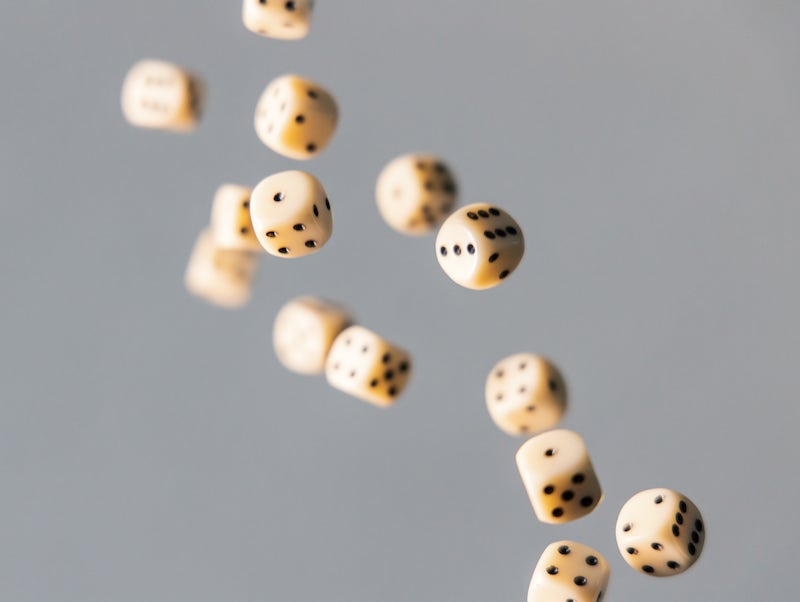# Probability vs Likelihood vs Maximum Likelihood

Theory and examples of Probability vs Likelihood vs Maximum Likelihood
Statistics
Machine Learning
Published

April 29, 2020# Probability

Probability is the quantitative measure of certainty of an event. Mathematically, probability $$P$$ for an event $$E$$ is defined as:

$$P(E) = \frac{number\ of\ times\ event\ E\ occurred}{Total\ number\ of\ events}$$

Consider a fair die, possible outcomes of rolling the die can be 1 to 6. Where probability of each outcome is 1/6. $$P(1) = P(2) = P(3) = P(4) = P(5) = P(6) = 1/6$$

What if we roll two dice together? What will be the probability that both dice will get the same digits, say 6?

$$P(dice_1=6\cap dice_2=6) = P(dice_1=6) \times P(dice_2=6)=\frac{1}{6} \times \frac{1}{6}$$

What if one of the dice is biased towards 6, i.e. $$P(dice_1=6) = 1/4\ and\ P(dice_2=6)=1/6$$

The probability will become $$P(dice_1=6\cap dice_2=6) = 1/6 \times 1/4$$

## Joint Probability

Joint probability is the probability of two events occurring simultaneously. Rolling two dice together is an example of Joint Probability.

If the two events E1 and E2 are independent of each other then the joint probability is multiplication of P(E1) and P(E2).

$$P(E_1\ and\ E_2) = P(E_1) \times P(E_2)$$

P(A given B) is denoted as P(A|B)

If the two events E1 and E2 are not independent then the joint probability is:

$$P(E_1\ and\ E_2) = P(E_1|E_2)P(E_2) = P(E_2|E_1)P(E_1)$$

### Joint probability is symmetric

P(A,B) = P(B, A)

P(A,B) = P(B|A)P(A)

P(B,A) = P(A|B)P(B)

P(A|B)P(B) = P(B|A)P(A)

$$P(A|B) = \frac{P(B|A)P(A)}{P(B)}$$

## Conditional Probability

It is the probability of one event when occurrence of the other is given.

Consider two events A and B, given that B has occurred. Then the probability of A given B is:

$$P(A\|B) = \frac{P(B\|A)P(B)}{P(A)} = \frac{P(A, B)}{P(A)}$$

## Maximum Likelihood

Maximum Likelihood is used to find the normal distribution of the given data. We estimate $$\mu$$ and $$\sigma$$ for the distribution.